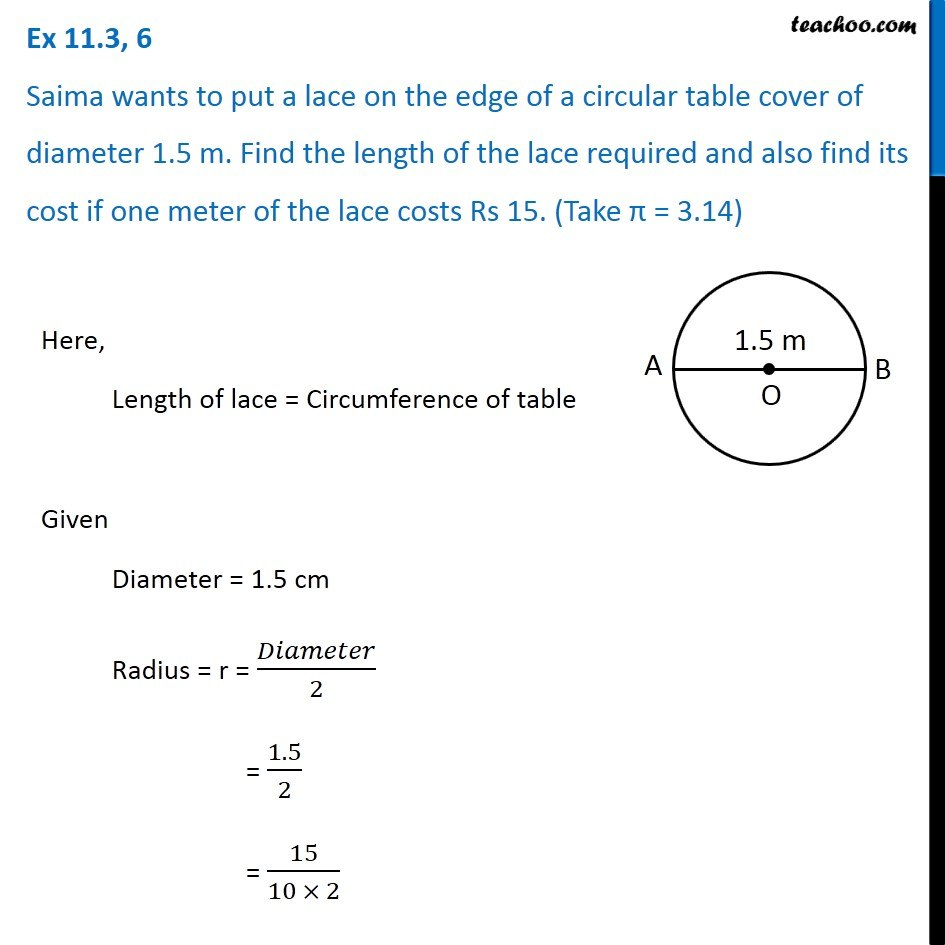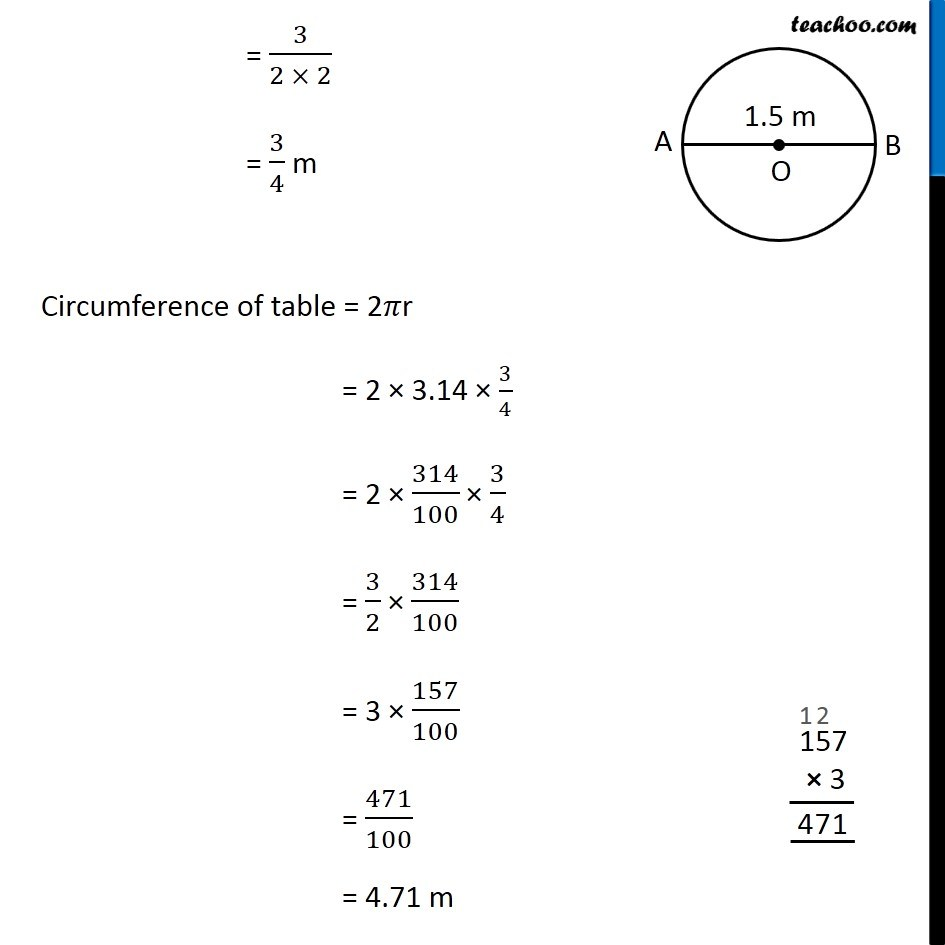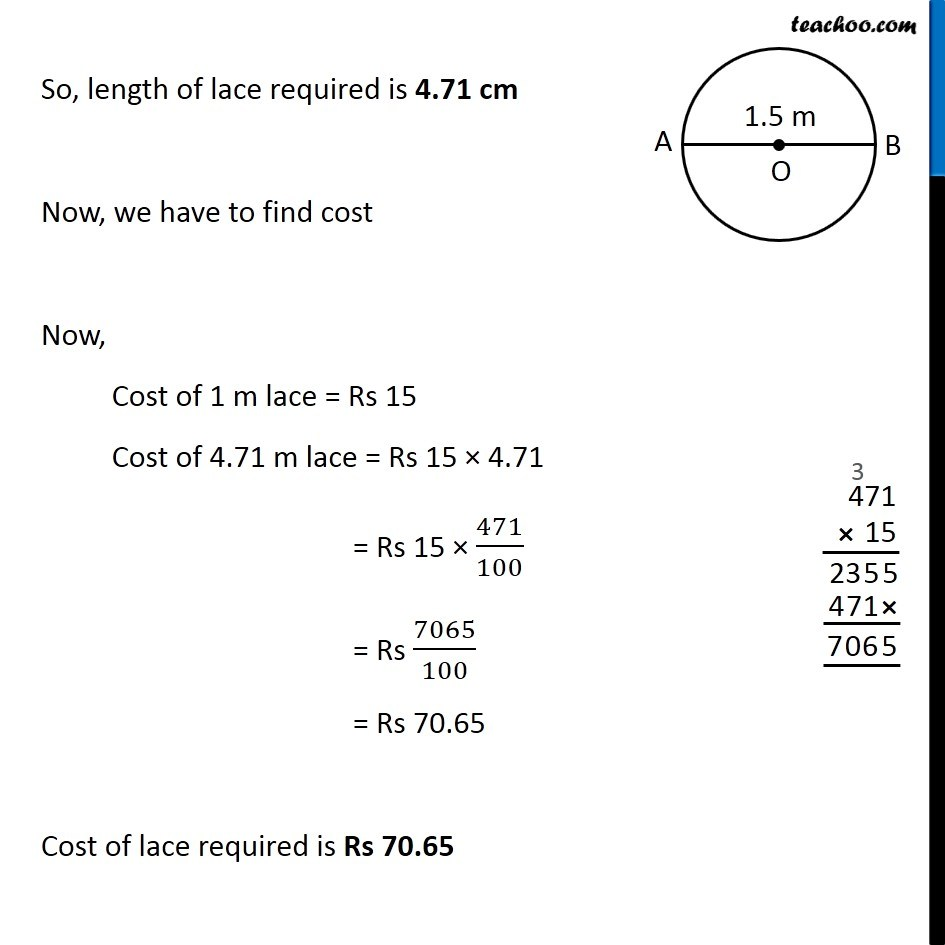1. Chapter 11 Class 7 Perimeter and Area
2. Concept wise
3. Perimeter of a combination of shapes

Transcript

Ex 11.3, 6 Saima wants to put a lace on the edge of a circular table cover of diameter 1.5 m. Find the length of the lace required and also find its cost if one meter of the lace costs Rs 15. (Take π = 3.14) Here, Length of lace = Circumference of table Given Diameter = 1.5 cm Radius = r = 𝐷𝑖𝑎𝑚𝑒𝑡𝑒𝑟/2 = 1.5/2 = 15/(10 × 2) = 3/(2 × 2) = 3/4 m Circumference of table = 2𝜋r = 2 × 3.14 × 3/4 = 2 × 314/100 × 3/4 = 3/2 × 314/100 = 3 × 157/100 = 471/100 = 4.71 m So, length of lace required is 4.71 cm Now, we have to find cost Now, Cost of 1 m lace = Rs 15 Cost of 4.71 m lace = Rs 15 × 4.71 = Rs 15 × 471/100 = Rs 7065/100 = Rs 70.65 Cost of lace required is Rs 70.65

Perimeter of a combination of shapes